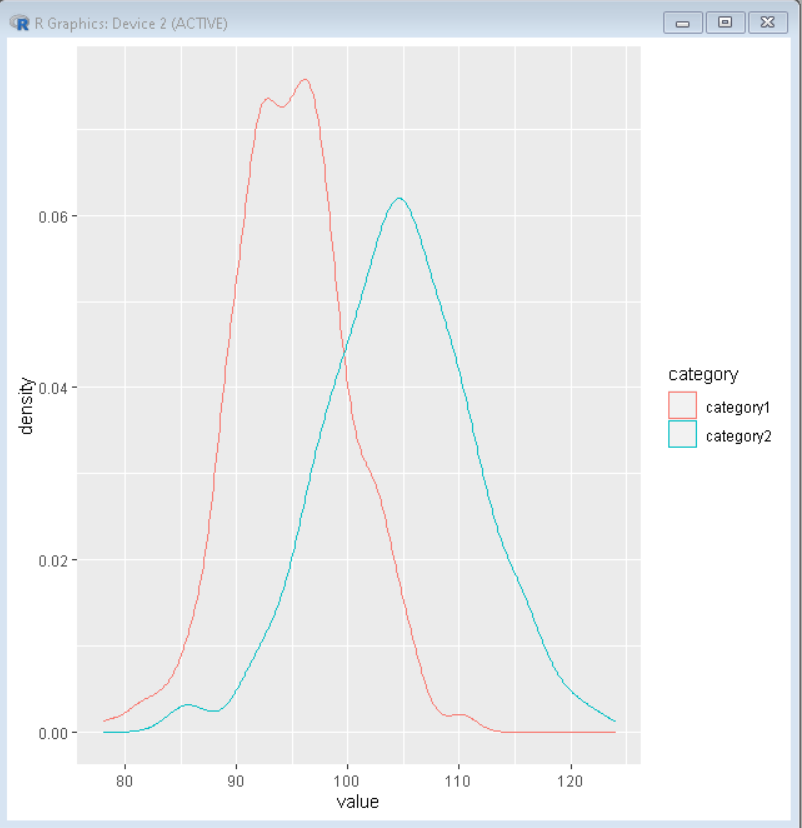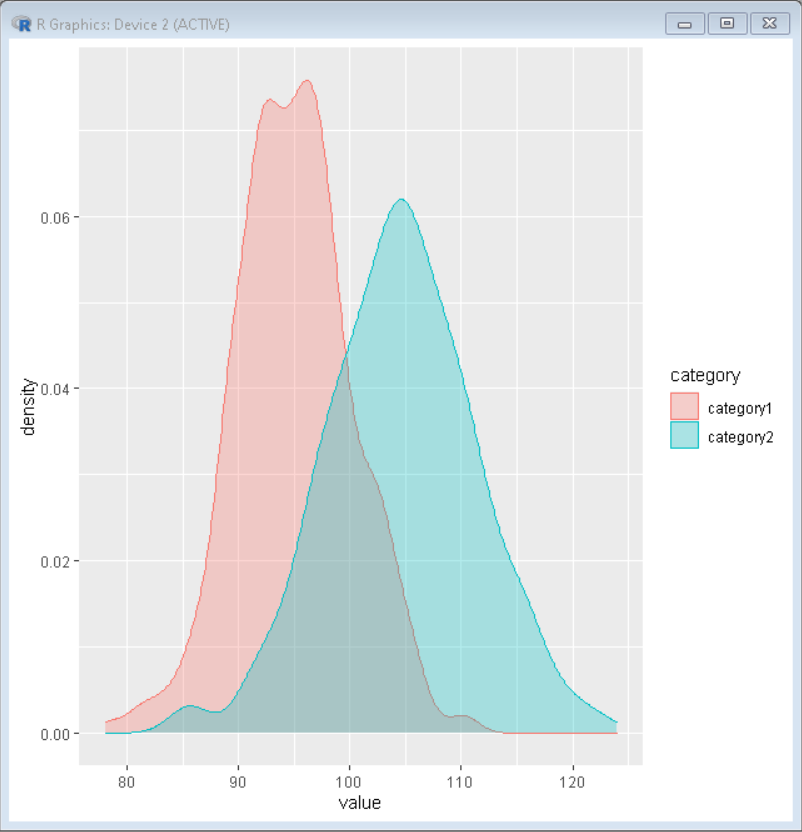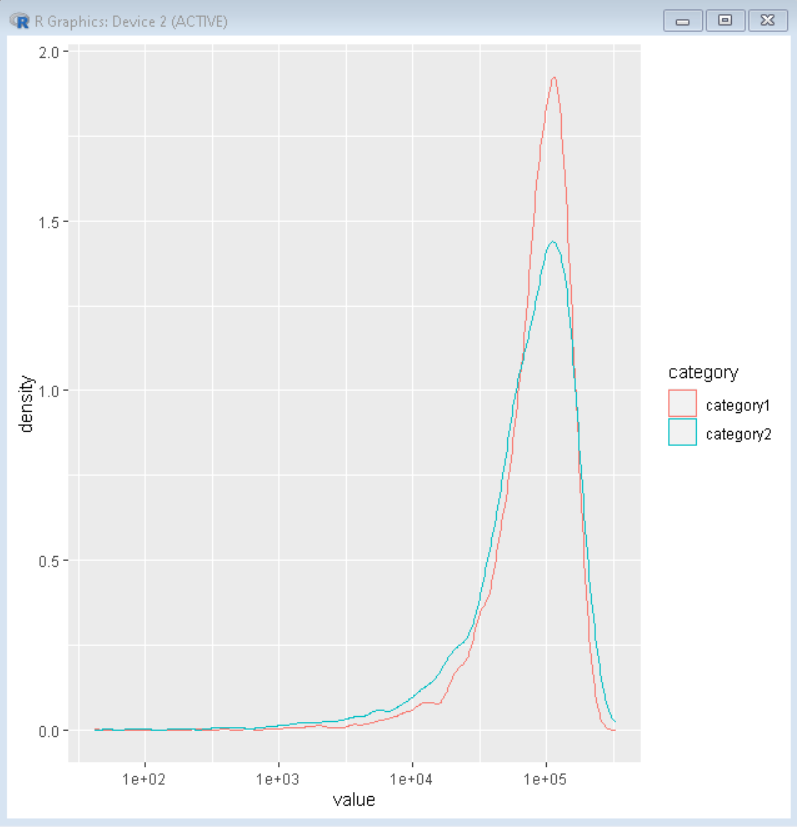Open in App
Not now

# Multiple Density Plots and Coloring by Variable with ggplot2 in R

• Last Updated : 05 Oct, 2021

In this article, we will discuss how to make multiple density plots with coloring by variable in R Programming Language.

To make multiple density plots with coloring by variable in R with ggplot2, we firstly make a data frame with values and category. Then we draw the ggplot2 density plot using the geom_desnity() function. To color them according to the variable we add the fill property as a category in ggplot() function.

Syntax: ggplot(dataFrame, aes( x, color, fill)) + geom_density()

## Multiple Density Plots with Coloring by Variable

We get multiple density plots in the ggplot of two colors corresponding to two-level/values for the second categorical variable. If our categorical variable has n levels, then ggplot2 would make multiple density plots with n densities/color.

## R

 `# set seed``set.seed``(1234)`` ` `# create dataframe``df <- ``data.frame``( category=``factor``(``rep``(``c``(``"category1"``, ``                                        ``"category2"``), ``                                      ``each=500)),``                    ``value=``round``(``c``(``rnorm``(500, mean=95, sd=5),``                                ``rnorm``(500, mean=105, sd=7))))``# load library ggplot2 package``library``(ggplot2)`` ` `# Basic density plot with custom color``ggplot``(df, ``aes``(x=value, color=category)) + `` ` `  ``# color property for changing color of plot``  ``# geom_density() function plots the density plot``  ``geom_density``()`

Output:## Color Customization

To add color beneath the density plot we use the fill property. We can also customize the transparency of the plot with the alpha property. We get multiple density plots in the ggplot filled with two colors corresponding to two-level/values for the second categorical variable. If our categorical variable has n levels, then ggplot2 would make multiple density plots with n densities/color. The alpha value of 0.3 gives 30% transparency to the plot fill.

## R

 `set.seed``(1234)``df < - ``data.frame``(``    ``category=``factor``(``rep``(``c``(``"category1"``, ``"category2"``), each=500)),``    ``value=``round``(``c``(``rnorm``(500, mean=95, sd=5),``                  ``rnorm``(500, mean=105, sd=7)))``)``# load library``library``(ggplot2)`` ` `# Basic density plot with custom color``# color property to determine the color of plot``# fill property to determine the color beneath plot``ggplot``(df, ``aes``(x=value, color=category, fill=category)) +``geom_density``(alpha=0.3)`

Output:## Log scale customization

When x or y-axis data is very large, we can draw plots with a log scale. In ggplot2, we can transform x-axis values to log scale using scale_x_log10() function.

Syntax: plot+ scale_x_log10()

Now our density plot is drawn in Log scale. This becomes more effective when our dataset is skew. It helps to correct the skewness of the plot.

## R

 `# set seed``set.seed``(1234)`` ` `# create dataframe``df < - ``data.frame``(``    ``category=``factor``(``rep``(``c``(``"category1"``, ``"category2"``),``                        ``each=5000)),``    ``value=``round``(``c``(``rnorm``(5000, mean=90005, sd=50000),``                  ``rnorm``(5000, mean=70005, sd=70000))))`` ` `# load library ggplot2``library``(ggplot2)`` ` `# Basic density plot``# color property is used to determine the color of plot``ggplot``(df, ``aes``(x=value, color=category)) +``geom_density``()+  ``# geom_density() creates density plot``scale_x_log10``()  ``# converts x-axis values in log scale`

Output:My Personal Notes arrow_drop_up